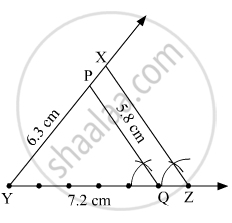Advertisement Remove all ads

# Construct ∆Pyq Such That, Py = 6.3 Cm, Yq = 7.2 Cm, Pq = 5.8 Cm. If Y Z Y Q = 6 5 , Then Construct ∆Xyz Similar to ∆Pyq. - Geometry

Sum

Construct ∆PYQ such that, PY = 6.3 cm, YQ = 7.2 cm, PQ = 5.8 cm. If $\frac{YZ}{YQ} = \frac{6}{5},$ then construct ∆XYZ similar to ∆PYQ.

Advertisement Remove all ads

#### Solution

Steps of Construction:
Step 1: Draw ∆PYQ such that PY = 6.3 cm, YQ = 7.2 cm and PQ = 5.8 cm.
Step 2: Divide segment YQ into 5 equal parts.
Step 3: Mark point Z on ray YQ such that length of YZ is six times of each part of segment YQ.
Step 4: Draw a line parallel to side QP, through Z. Name the point where the parallel line intersects ray YP as X.Here, ∆PYQ ~ ∆XYZ.

Is there an error in this question or solution?
Advertisement Remove all ads

#### APPEARS IN

Balbharati Mathematics 2 Geometry 10th Standard SSC Maharashtra State Board
Chapter 4 Geometric Constructions
Problem Set 4 | Q 8 | Page 99
Advertisement Remove all ads
Advertisement Remove all ads
Share
Notifications

View all notifications